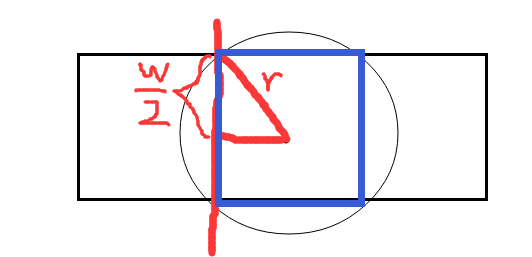## 喷水装置问题 贪心算法``````3
8 20 2
5 3
4 1
1 2
7 2
10 2
13 3
16 2
19 4
3 10 1
3 5
9 3
6 1
3 10 1
5 3
1 1
9 1
``````

``````6
2
-1
````````````#include <iostream>
#include<bits/stdc++.h>
using namespace std;
int t,n,l;//组数，喷头数，长度
int s;//喷头位置
double w,r;//草坪宽度，喷头半径
double maxn;//临时变量
int main()
{

vector< pair<double,double> >vec;
pair<double,double> p;
cin>>t;//输入总组数
while(t--)//t--不等于0时
{

cin>>n>>l>>w;//输入喷头数，草坪长、宽
vec.clear();//确保容器vec为空
for(int i=0;i<n;i++)
{

cin>>s>>r;//输入喷头位置、半径
if(r<w/2)
continue;//如果半径小于宽度的一半，则一定不会覆盖草坪，获取下一个喷头数据
r=sqrt(r*r-(w/2*w/2));//勾股定理逆用,
p=make_pair(s-r,s+r);//求区间
vec.push_back(p);//尾部插入区间
}
sort(vec.begin(),vec.end());//升序
int k=0;//记录已遍历到的喷头数
double now=0;//记录覆盖到的位置
int ans=0;//记录选用的的喷头数
int flag=1;//标记是否还能覆盖
int len=vec.size();//容器大小
while(k<len)
{

if(now<vec[k].first)
{

flag=0;
break;}//前面覆盖到的位置与当前可覆盖区间不相交，即存在不覆盖的情况
if(now>=l)break;//覆盖已完成
maxn=-1;
while(now>=vec[k].first&&k<len)//当下一个区间可覆盖，尽可能选覆盖区间最大的
{

maxn=max(maxn,vec[k].second);
k++;//记录已遍历的喷头数
}
now=maxn;
ans++;//每换一个区间，选用的喷头数加一
}
if(flag==1)
cout<<ans<<endl;
else
cout<<"-1"<<endl;
}
return 0;
}
``````